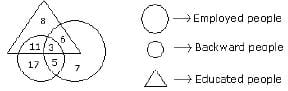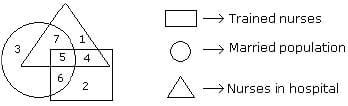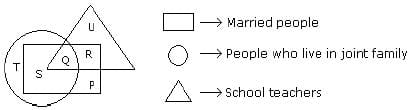# Olympiad Test: Venn Diagrams -2

Test Description

## 20 Questions MCQ Test Science Olympiad Class 7 | Olympiad Test: Venn Diagrams -2

Olympiad Test: Venn Diagrams -2 for Class 7 2023 is part of Science Olympiad Class 7 preparation. The Olympiad Test: Venn Diagrams -2 questions and answers have been prepared according to the Class 7 exam syllabus.The Olympiad Test: Venn Diagrams -2 MCQs are made for Class 7 2023 Exam. Find important definitions, questions, notes, meanings, examples, exercises, MCQs and online tests for Olympiad Test: Venn Diagrams -2 below.
 1 Crore+ students have signed up on EduRev. Have you?
Olympiad Test: Venn Diagrams -2 - Question 1

### Directions: Each of these questions given below contains three elements. These elements may or may not have some inter linkage. Each group of elements may fit into one of these diagrams at (a), (b), (c), (d). You have to indicate the group of elements which correctly fits into the diagrams. Q. Which of the following diagrams indicates the best relation among Class, Blackboard and School?

Detailed Solution for Olympiad Test: Venn Diagrams -2 - Question 1

Blackboard is in class and class is in the school.

Olympiad Test: Venn Diagrams -2 - Question 2

### Directions: Each of these questions given below contains three elements. These elements may or may not have some inter linkage. Each group of elements may fit into one of these diagrams at (a), (b), (c), (d). You have to indicate the group of elements which correctly fits into the diagrams. Q. Which of the following diagrams indicates the best relation among Rabi-Crop, Paddy and Wheat?

Detailed Solution for Olympiad Test: Venn Diagrams -2 - Question 2

Wheat and Paddy are different from each other but Wheat is the Rabi-Crop.

Olympiad Test: Venn Diagrams -2 - Question 3

### Directions: Each of these questions given below contains three elements. These elements may or may not have some inter linkage. Each group of elements may fit into one of these diagrams at (a), (b), (c), (d). You have to indicate the group of elements which correctly fits into the diagrams. Q. Which of the following diagrams indicates the best relation among Hospital, Nurse and Patient?

Detailed Solution for Olympiad Test: Venn Diagrams -2 - Question 3

Hospital consists of nurse and patient but nurse and patient are of two different nature.

Olympiad Test: Venn Diagrams -2 - Question 4

Directions: Each of these questions given below contains three elements. These elements may or may not have some inter linkage. Each group of elements may fit into one of these diagrams at (a), (b), (c), (d). You have to indicate the group of elements which correctly fits into the diagrams.

Q. Which of the following diagrams indicates the best relation among Mercury, Zinc and Metal?

Detailed Solution for Olympiad Test: Venn Diagrams -2 - Question 4

Mercury and Zinc both are different from each other but belong to metal.

Olympiad Test: Venn Diagrams -2 - Question 5

Directions: Each of these questions given below contains three elements. These elements may or may not have some inter linkage. Each group of elements may fit into one of these diagrams at (a), (b), (c), (d). You have to indicate the group of elements which correctly fits into the diagrams.

Q. Which of the following diagrams indicates the best relation among Teacher, Writer and Musician?

Detailed Solution for Olympiad Test: Venn Diagrams -2 - Question 5

A teacher may or may not be a writer and musician. Similarly a musician may or may not be a teacher and writer and so a writer may or may not be a teacher and musician.

Olympiad Test: Venn Diagrams -2 - Question 6

Directions: Each of these questions given below contains three elements. These elements may or may not have some inter linkage. Each group of elements may fit into one of these diagrams at (a), (b), (c), (d). You have to indicate the group of elements which correctly fits into the diagrams.

Q. Which of the following diagrams indicates the best relation among Iron, Lead and Nitrogen?

Detailed Solution for Olympiad Test: Venn Diagrams -2 - Question 6

All the three elements are different from each other.

Olympiad Test: Venn Diagrams -2 - Question 7

Directions: Each of these questions given below contains three elements. These elements may or may not have some inter linkage. Each group of elements may fit into one of these diagrams at (a), (b), (c), (d). You have to indicate the group of elements which correctly fits into the diagrams.

Q. Which of the following diagrams indicates the best relation among Examination, Questions and Practice?

Detailed Solution for Olympiad Test: Venn Diagrams -2 - Question 7

Some questions are asked in examination and some in practice but examination and practice are different from each other.

Olympiad Test: Venn Diagrams -2 - Question 8

Directions: Each of these questions given below contains three elements. These elements may or may not have some inter linkage. Each group of elements may fit into one of these diagrams at (a), (b), (c), (d). You have to indicate the group of elements which correctly fits into the diagrams.

Q. Which of the following diagrams indicates the best relation among Bulb, Lamp and Light?

Detailed Solution for Olympiad Test: Venn Diagrams -2 - Question 8

Bulb and Lamp are different from each other but light is obtained from both.

Olympiad Test: Venn Diagrams -2 - Question 9

Directions: Each of these questions given below contains three elements. These elements may or may not have some inter linkage. Each group of elements may fit into one of these diagrams at (a), (b), (c), (d). You have to indicate the group of elements which correctly fits into the diagrams.

Q. Which of the following diagrams indicates the best relation among Lion, Dog and Snake?

Detailed Solution for Olympiad Test: Venn Diagrams -2 - Question 9

All the three are different from each other.

Olympiad Test: Venn Diagrams -2 - Question 10

Directions: Each of these questions given below contains three elements. These elements may or may not have some inter linkage. Each group of elements may fit into one of these diagrams at (a), (b), (c), (d). You have to indicate the group of elements which correctly fits into the diagrams.

Q. Which of the following diagrams indicates the best relation among Moon, Sun and Earth?

Detailed Solution for Olympiad Test: Venn Diagrams -2 - Question 10

All the three are different from each other.

Olympiad Test: Venn Diagrams -2 - Question 11

Directions: Study the following figure and answer the questions given below.Q. How many educated people are employed?

Detailed Solution for Olympiad Test: Venn Diagrams -2 - Question 11

Number of educated people who are employed = 3 + 6 = 9.

Olympiad Test: Venn Diagrams -2 - Question 12

Directions: Study the following figure and answer the questions given below.Q. How many backward people are educated?

Detailed Solution for Olympiad Test: Venn Diagrams -2 - Question 12

Number of backward people are who are educated = 11 + 3 = 14.

Olympiad Test: Venn Diagrams -2 - Question 13

Directions: Study the following figure and answer the questions given below.Q. How many backward uneducated people are employed?

Detailed Solution for Olympiad Test: Venn Diagrams -2 - Question 13

Number of backward uneducated people who are employed is 5.

Olympiad Test: Venn Diagrams -2 - Question 14

Directions: Study the following figure and answer the questions given below.Q. How many backward people are not educated?

Detailed Solution for Olympiad Test: Venn Diagrams -2 - Question 14

Number of backward people who are not educated = 17 + 5 = 22.

Olympiad Test: Venn Diagrams -2 - Question 15

Directions: Study the following figure and answer the questions given below.Q. If the hospital management requires only married trained nurses for operation theatre, which number of diagram should be chosen by it?

Olympiad Test: Venn Diagrams -2 - Question 16

Directions: Study the following figure and answer the questions given below.Q. By which number, married but untrained nurses in the hospital are represented?

Olympiad Test: Venn Diagrams -2 - Question 17

Directions: Study the following figure and answer the questions given below.Q. By which numbers trained nurses are represented?

Olympiad Test: Venn Diagrams -2 - Question 18

Directions: Study the following figure and answer the questions given below.Q. What is represented by the number 7?

Olympiad Test: Venn Diagrams -2 - Question 19

Directions: Study the following figure and answer the questions given below.Q. By which letter, the married teachers who live in joint family are represented?

Olympiad Test: Venn Diagrams -2 - Question 20

Directions: Study the following figure and answer the questions given below.Q. By which letter, the married people who live in joint family but not are school teachers are represented?